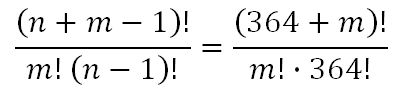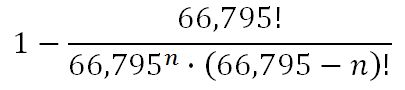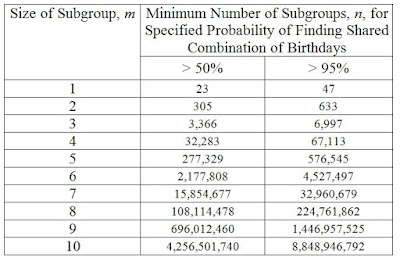## Saturday 10 October 2015

### A Fistful of BirthdaysWhat? Well, it can't be worse than Paint Your Wagon.

To commemorate my birthday, I'm going to solve yet another variation of the Birthday Problem. So far we've looked at the probability of any two people in a group having the same birthday, the probability of having a "near match", the probability of the first match or near-match as people join a group one at a time, and probability of matching or nearly matching a specific birthday (such as your own).

Suppose instead of looking to match any pair of individual birthdays, you want to match a pair of combinations of birthdays, like couples' birthdays or entire families' birthdays. For instance, how unusual would it be to discover that a couple has matching birthdays with another couple at a party?

Let's start with a little review from the classic birthday problem. What are the chances that two randomly selected people have the same birthday? Assuming that birthdays are evenly distributed among the 365 calendar days (let's neglect those leap-year birthdays) and everyone's birthdays are independent of each other, the probability is 1/365 (about 0.27%).

Well what about matching couples birthdays? We start by calculating the number of different possible combinations of birthdays that exist for two randomly selected people. Using the formula for combinations with repetition (Equation 1), where n = 365 days and m = 2 people, I calculate there are 66,795 possible combinations for a random couple. So there's a 1 in 66,795 (about 0.0015%) chance that two randomly selected couples will share the same birthdays between them.Equation 1

This assumes that the order is not important; it is only necessary that the same birthdays be shared by both groups. For example, A1 & A2 are born January 3rd and February 5th. B1 & B2 would be considered a match if B1 is born January 3rd and B2 is born February 5th. B1 & B2 would also be considered a match if B2 is born January 3rd and B1 is born February 5th.

If there's a 1 in 66,795 that two couples have matching combinations of birthdays, that means there's a 66,794/66,795 probability that two couples don't have matching combinations of birthdays. Having taken up two combinations of birthdays with couples A and B means that a third couple, C, has a 66,793/66,795 chance of not sharing their combination of birthdays with either A or B. The probability of no shared combination of birthdays in the group of three couples is [66,794/66,795] * [66,793/66,795] (about 99.9955%). To find the probability of at least one successful match, simply subtract the probability of no shared birthdays from 100%. So about 0.0045% chance of a shared combination of birthdays among three randomly selected couples. Generalizing now to n randomly selected couples, we get this complicated-looking formula:Equation 2: Probability of at least one shared combination of birthdays among n randomly selected couples.

While Equation 2 is correct, you're dealing with huge numbers that typical calculators simply can't handle, so it'd be good to have a different version of this expression. Using pi notation (product of a sequence), we can rewrite Equation 2 to get a version that's computationally easier to work with:Equation 3: Alternate expression of Equation 2

And with that, we can crunch the numbers to figure out how many random couples you need to invite to the party to have a reasonable chance of a matching combination of birthdays.Minimum number of couples required for a specified probability of at least one pair of couples with matching birthday combinations.

With 13 couples you have a little less than 0.12% chance of a match. You need 38 couples before there's at least 1% chance of a match, and 120 couples to have a 1 in 10 chance. At least one matching combination becomes more likely than not if there are at least 305 couples. There's less than 10% chance of not having a match if there are more than 555 couples. At 1,355 couples, you're practically guaranteed (better than 99.9999% probability) at least one match. Below is a pretty graph from n = just 1 couple (guaranteed no match since there are no other couples) all the way up to 66,796 couples (guaranteed to have at least one match by the pigeonhole principle).Figure 1: Probability of at least one pair of couples with matching birthday combinations in a group of n randomly selected couples. Note: horizontal axis is plotted on logarithmic scale.In case you were wondering, this analysis doesn't apply to groups of twins.
So the probability of finding at least two couples with matching birthday combinations is pretty unlikely in common scenarios like a dancing class or the office Christmas party, but there could be a reasonable chance at very large gatherings like a traditional Mediterranean wedding, and it's practically guaranteed at stadium-sized events like a concert or professional hockey game.

What if we want to know about matching birthday combinations involving a larger grouping, like a family of 4? Well if we go back to Equation 3 and replace the value 66,795 with the right-hand side of Equation 1, we'll have a general expression to calculate the probability that, among n randomly selected subgroups of m people, there's at least one pair of them having matching birthday combinations. That general formula looks like this:Equation 4: General expression to find the probability of at least one pair of m-sized subgroups with matching birthday combinations among n subgroups.

So if you're interested in the probability of at least one pair of matching combinations of birthdays among 25,000 randomly selected families of 4, set m = 4 and n = 25,000 (p  34.0%). I've expanded the previous plot for m = 3, m = 4, and m = 6.Figure 2: Probability of at least one pair of m-sized subgroups with matching birthday combinationsin a group of n randomly selected subgroups.

As you probably guessed, as m increases, you need an increasingly large number of n subgroups to get to a reasonable probability of a match. It also quickly gets too difficult to solve with a personal computer unless you start using a more manageable numerical approximation. For m = 7 and up I switched to using Ramanujan's approximation of the factorial function (which is quite accurate) and used logarithms to reduce the size of the numbers involved.By taking logarithms and using Ramanujan's approximation, the problem of solving for p begins to look more complicated, but is actually much easier for your computer to handle.

Fun fact: using logarithms simplifies math problems because exponentiation reduces to multiplication, multiplication reduces to addition, very large numbers become much smaller, and all the numbers you're working with become more similar in size. Before calculators and computers, most scientists and engineers were quite familiar with this trick and regularly referred to printed handbooks containing tables of logarithms in order to complete their calculations.Equation 5: Calculate p after you've found the approximate solution to ln q.
I used the above approximations to calculate ln q and the false position method to find the minimum value of required to have either a 50% or 95% probability of finding a matching pair:Minimum number n for 50% or 95% probability of a matching pair of birthday combinations for m-sized subgroups

I also decided to work out what's the largest subgroup size m where we would have a reasonable chance of finding a matching combination of birthdays in another subgroup, assuming all subgroups are selected from the entire population of Earth. Set n = 7,300,000,000/m, vary m, and solve for the probability of finding at least one matching pair on Earth.

Just based on the pattern observed in Figure 2, I was able to extrapolate and guess that the largest subgroup size m would probably be 9 (there's almost an order of magnitude increase in n to get to the steeply sloped part of the curve as m increases by 1). In fact, for m = 8 and n = 912,500,000 random groups of 8, the probability of a matching pair of birthday combinations is infinitesimally close to a mathematical guarantee (slightly more than 99.999999999999999999964%). For m = 9 and n = 811,111,111 random groups of 9, the probability of a matching pair of birthday combinations is just over 60.99%. For m = 10 and n = 730,000,000, the probability of finding a matching pair of birthday combinations is a little under 2.02%. At m = 11 and n = 663,636,364, the probability of finding a match is about 1 in 2,024.

In summary:  Finding a pair of matching individual birthdays at the office: fairly likely. Matching couples' birthdays at a party? Maybe, but it'd probably need to be a pretty large party. Matching the combination of birthdays in a group of 10 or more? Nearly impossible, even if the whole planet is involved.